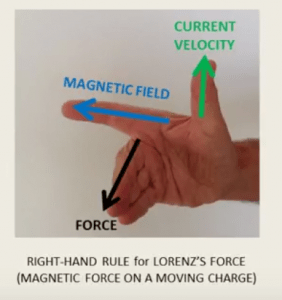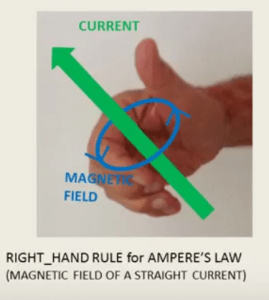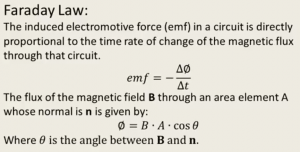# Bypassing Lenz’s Rule

Here is another example of an interesting physics topic, which is not included in the book due to its limited relevancy to architecture. (based on an article in “The Physics Teacher” by the author).

## A left hand rule for Faraday’s Law

In electromagnetism, we have to deal with relationships between vectors in three dimensions. We quite often resort to hand rules that help us visualize the relationships between such vectors in various situations.

One right hand rule describes the relationship between the directions of the velocity of a charged particle, the direction of the magnetic field (in which that particle moves), and the resulting force that acts on that particle (Lorentz’s Force).Another right hand rule describes the relationship between the direction of a current and the magnetic field that it generates (Ampere’s Law).## A new hand rule

### A Left Hand Rule for Faraday’s Law

Faraday’s Law deals with the relationships between the change in magnetic flux and the induced electromotive force. Magnetic flux combines in it two vectors: magnetic field and a normal to a surface enclosed by a loop. The induced electromotive force includes in it an electric field and the directions of elements of a loop.In order to find the relationships between the directions of all those vectors, one usually applies Lenz’s Law: “The direction of the induced emf is such that the current that it drives creates a magnetic field that opposes the change that has caused the induced emf”.

It is now possible to apply a new left hand rule and bypass the need to apply Lenz’s Law.• Align the curved fingers of the left hand with the loop (yellow line).
• The stretched thumb points to the direction of the normal to the surface enclosed by the loop (brown).
• The magnetic field is shown by the red arrow.
• Find the change in flux using this normal (n) and magnetic field (B).
• If the change in flux is positive, the curved fingers show the direction of the induced electromotive force (yellow arrows).
• If the change in flux is negative, the direction of the induced electromotive force is opposite to the direction of the curved fingers (opposite to the yellow arrows).

#### ExampleA circular conductor (blue) is placed in a magnetic field that points towards us and decreases with time. In order to find the induced electromotive force in the loop we set the left hand as shown in the figure. The magnetic field (B) happens to point in this case in the thumb’s direction, which is the direction of the normal (n). The angle between B and n is zero and the cosine is one. Therefore, both the initial and final fluxes are positive. Since the magnetic field is decreasing with time, the change in flux is negative. According to the new left hand rule, the direction of the induced electric field is against the curved fingers, as shown by the curved arrows (counter clockwise).

Comment: We could have set the left hand so that the thumb points in the opposite direction (into the page). In that case, both initial and final fluxes would be negative (cos 180°=-1) and their difference would be positive. Therefore the emf would point in the direction of the curved fingers, which is again as shown by the curved arrows, because now the fingers are curved opposite to those in the figure.

## The justification for the new left hand rule

(You don’t have to know this in order to use the new left hand rule…)